# Continuation method (to a parametrized family)

The inclusion of a given problem in a one-parameter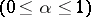family of problems, connecting the given problemwith a problem that is known to be solvable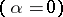, and the study of the dependence of solutions on the parameter. The method is extensively used in the theory of differential equations.

Suppose, e.g., that one has to prove the solvability in a Hölder class of the Dirichlet problem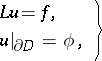(1)

in a suitable bounded-dimensional regionfor the linear elliptic second-order operator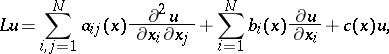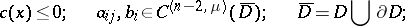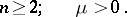One introduces the family of elliptic operators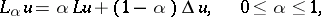and considers for it the Dirichlet problem(2)

Letbe the set of allfor which (2) is uniquely solvable infor any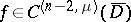and. The setis not empty, since for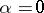(i.e. for the Laplace operator) (2) is uniquely solvable in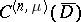, which follows from potential theory. The setis at the same time open and closed in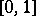, hence coincides with it. Thus,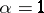belongs toand (1) is solvable.

The continuation method (in the case of analytic continuation) was proposed and developed in a number of papers by S.N. Bernstein [S.N. Bernshtein], cf. , . Subsequently, this method gained wide application in various problems in the theory of linear and non-linear differential equations, where the idea of analytic continuation was supplemented by more general functional and topological principles (cf. ).

How to Cite This Entry:
Continuation method (to a parametrized family). I.A. Shishmarev (originator), Encyclopedia of Mathematics. URL: http://encyclopediaofmath.org/index.php?title=Continuation_method_(to_a_parametrized_family)&oldid=18507
This text originally appeared in Encyclopedia of Mathematics - ISBN 1402006098